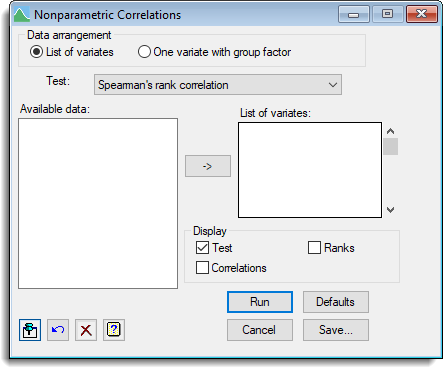1. Home
2. Nonparametric Correlations

# Nonparametric Correlations

Select menu: Stats | Statistical Tests | Spearman’s Rank Correlation or Kendall’s Rank Correlation Coefficient

Use this to calculate nonparametric correlations between pairs of samples. There are two methods available; Spearman’s rank correlation and Kendall’s rank correlation coefficient.

Stats | Statistical Tests | Nonparametric Correlations then select one of the two methods.
2. Fill in the fields and elect to Save the results if required, then click Run.Spearman’s rank correlation coefficient is a measure of association between the rankings of two variables measured on N individuals (i.e. two vectors of length N). The correlation coefficient is calculated from the vectors of ranks for each pair of samples.

Kendall’s rank correlation coefficient, tau, is a measure of association between the rankings of two variables measured on N individuals. It is calculated as S / SQRT(NC1 * NC2). S is defined as the sum of SIGN(Xi – Xj) * SIGN(Yi – Yj)) over all pairs of distinct units i and j. NC1 and NC2 are the number of valid comparisons (removing ties and missing values) that can be made amongst the first and second set of samples, respectively.

## Data arrangement

You can supply the data either as a list of variates or as a single variate with a factor defining the groups.

 List of Variates The samples must be supplied as a list of variates, whose names should be entered in the List of variates field One variate with group factor The data must be supplied in one variate, specified as the Data variate. Membership of the different samples is then indicated by the Group factor.

## Test

Controls the type of nonparametric correlation test to be used.

## Available data

List variates and factors that can be used to supply the data sets and groups. The contents will change as you move from one input field to another, so that appropriate types of data structure are listed. You can double-click a name to copy it to the current input field or type it in.

## Display

Specifies the output to be displayed.

 Test Correlation coefficient/matrix and relevant test statistics (Spearman rank correlation only). Probabilities Probability for correlation coefficient (Kendall’s tau only). Correlations Rank correlation coefficient. Ranks Ranks for each sample (but only for Spearman’s rank correlation).

## Action IconsPin Controls whether to keep the dialog open when you click Run. When the pin is downthe dialog will remain open, otherwise when the pin is upthe dialog will close.Restore Restore names into edit fields and default settings.Clear Clear all fields and list boxes.Help Open the Help topic for this dialog.